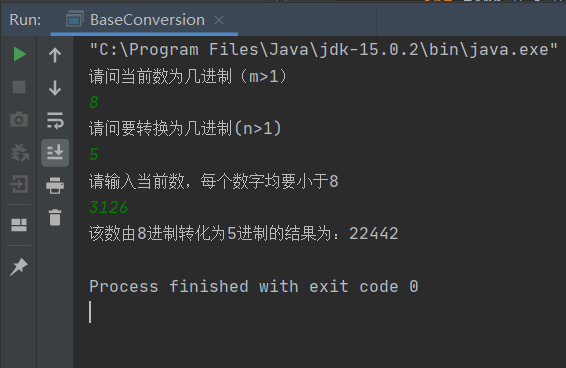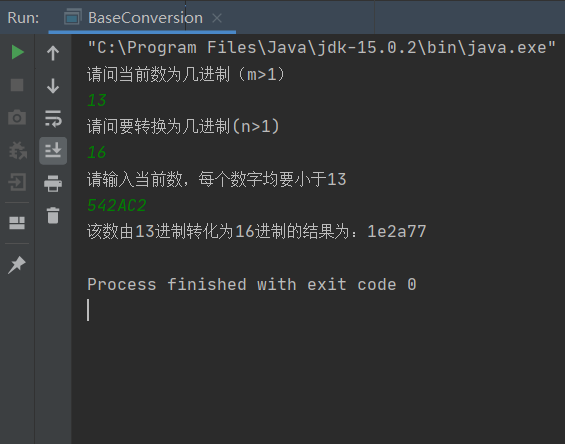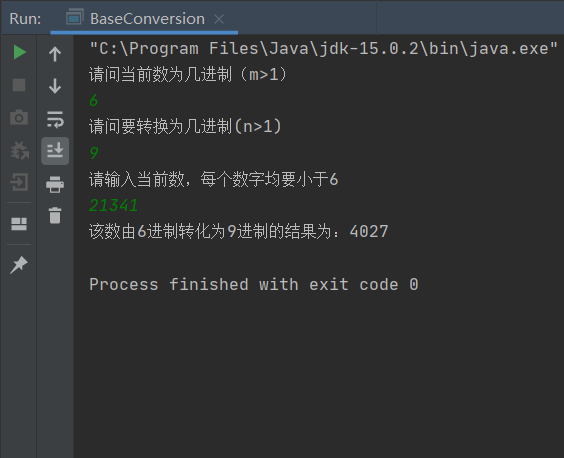• java桌面小应用程序 任意进制转换任意进制，包含从字符、字符串 转换为其他进制数
• JAVA实现蓝桥杯基础练习十六进制转换八进制-----------拓展进制转换计算器 这个题目我浏览了大量的道友的文章发现十分麻烦，但仍然从其中收益菲薄，所以在这里分享一下我认为最好的代码 import java.math.BigInteger...
JAVA实现蓝桥杯基础练习十六进制转换八进制-----------拓展进制转换计算器这个题目我浏览了大量的道友的文章发现十分麻烦，但仍然从其中收益菲薄，所以在这里分享一下我认为最好的代码
import java.math.BigInteger;
import java.util.Scanner;

public class L2021java_prictise {
public static void main(String[] args) {
Scanner scanner = new Scanner(System.in);
int n = scanner.nextInt();
BigInteger []a = new BigInteger[n];
for (int i = 0;i < n;i++){
a[i] = new BigInteger(scanner.next(),16);
}
for(int j = 0;j < n;j++){
System.out.print(a[j].toString(8)+"\n");
}
}
}

进阶版本的任意进制转换计算器：
import java.math.BigInteger;
import java.util.Scanner;

public class L2021java_prictise {
public static void main(String[] args) {
Scanner scanner = new Scanner(System.in);
System.out.print("请输入被转换数个数："+"\n");
int n = scanner.nextInt();
System.out.print("请输入被转换数进制数："+"\n");
int m = scanner.nextInt();
System.out.print("请输入将要转为____进制？："+"\n");
int t = scanner.nextInt();
System.out.print("请输入你要转换的数字以回车分隔："+"\n");
BigInteger []a = new BigInteger[n];
for (int i = 0;i < n;i++){
a[i] = new BigInteger(scanner.next(),m);
}
for(int j = 0;j < n;j++){
System.out.print(a[j].toString(t)+"\n");
}
}
}

希望对大家有所帮助，求赞！！！！
展开全文编程语言
• 进制与十进制互转，十以下进制任意转换。简单、没啥难度，就是写个样例以后可以直接copy。
	//十进制转二进制
public static String dec2Bin(int a){
String result="";
if(a==0)return "0";
while(a!=1){
if(a%2==0)result+="0";
else result+="1";
a/=2;
}//辗转相除
result+="1";
String reString="";
//倒序
for(int i=result.length()-1;i>=0;i--){
reString+=result.charAt(i);
}
return reString;
}

//二进制转十进制
public static int bin2Dec(String a){
int dec=0;
for(int i=0;i<a.length();i++){
int c=(int)(a.charAt(i)-'0');
dec+=c*pow(2,a.length()-i-1);
}
return dec;
}

//十进制以下进制任意转换(a进制数src，转b进制数),其实就是以上的两个算法的综合
public static String d2d(String src,int a,int b){
int sum=0;
for(int i=0;i<src.length();i++){
int c=(int)(src.charAt(i)-'0');
sum+=c*pow(a,src.length()-i-1);
}
String result="";
if(sum==0)return "0";
while(sum!=0){
int in=sum%b;
result+=Integer.toString(in);
sum/=b;
}
String reString="";
for(int i=result.length()-1;i>=0;i--){
reString+=result.charAt(i);
}
return reString;
}
其中，求幂函数可以用快速幂：

	//快速幂
public static int pow(int a,int b){
while(b!=0){
base*=base;
b>>=1;
}
}


展开全文string 实例 二进制
• (一)进制转换思想 先把任意进制转化为十进制 再把十进制转化为任意进制 本算法结合了十以下进制利用公式转换以及十以上进制调用函数转换 (二)问题分析 1.输入当前数进制 输入当前进制 m ，且保证 m>1 public ...
问题描述
编写程序实现任意进制间的相互转换

目录
问题描述(一)进制转换思想(二)问题分析1.输入当前数进制2.输入当前数4.输入待转化进制5.输出转化后数值
(三)完整代码(四)运行结果

(一)进制转换思想
先把任意进制转化为十进制再把十进制转化为任意进制本算法结合了十以下进制利用公式转换以及十以上进制调用函数转换
(二)问题分析
1.输入当前数进制
输入当前进制 m ，且保证 m>1
	public static void main (String[] args){
int l,m,n;
String l16;
Scanner sc = new Scanner(System.in);
System.out.println("请问当前数为几进制（m>1）");
m = sc.nextInt();

2.输入当前数
（1）m进制数要保证每个数字小于 m
		System.out.println("请输入当前数，每个数字均要小于"+m);
int temp=0,i=0;

（2）对于十以下进制，输入的是数值型，采用 l=sc.nextInt(); （3）利用公式计算转化为十进制，可调用 Math 的 pow(m，i) 函数，用以计算 m 的 i 次幂；数值的每个数字从右到左依次与 m^i （i=0,1，2……）相乘，可利用除10求余(%)运算；每计算一次，原数据缩小十倍且只保留整数部分(除运算 ：/ )，直到数值为0结束
        if(m<=10){
l=sc.nextInt();
while(l!=0){
temp+=(l%10)*Math.pow(m,i);
i+=1;
l=l/10;
}
}

（4）对于大于十的进制，输入的是字符串型，采用 l16=sc.next(); （5）可以直接调用 把任意进制转化为十进制 的包Integer.valueOf()
	else{
l16=sc.next();
temp=Integer.valueOf(l16,m);
}

（6）所以实际上这里面其实只用 temp=Integer.valueOf(l16,m); 就可以解决整个问题，因为十以下进制的数也能看成字符串型，这里为了介绍两种方法
4.输入待转化进制
这里仍然介绍两种方法，十以下进制、十以上进制分开处理
		System.out.println("请问要转换为几进制(n>1)");
n = sc.nextInt();

（1）十以下进制采用公式，并把该处理方法封装在一个类(ConversionN)里 （2）temp 为上一段转化后的十进制数，n 为待转进制 （3）十进制 temp 转化为 n 进制的思想是：当前数除 n 取余(% 运算)，得到的数字放在右边第一位（相当于该数字乘1）；把该数缩小十倍保留整数部分，继续除 n 取余，得到的数字放在右边第二位（相当于该数字乘10）；依次循环，把最终得到的数加在一起，就是转化的 n 进制数
	public static int ConversionN(int temp,int n){
int l=0,j=1;
while(n<=10 && temp!=0){
l+=(temp%n)*j;
temp=temp/n;
j=j*10;
}

return l;
}

（4）十以上进制直接调用 把十进制转换成任意进制 的包 Integer.toString()
		String L16=Integer.toString(temp,n);
System.out.println("该数由"+m+"进制转化为"+n+"进制的结果为："+L16);

5.输出转化后数值
（1）由于选择转化的进制不同（十以下，十以上），所以输出的路径也不同 （2）采用三目运算符根据范围选择路径：十进制以下路径1，十进制以上路径2，n <=1 路径3（提示输入有误） （3）结合 Switch 语句，完成选择语句
		int a = n<=10 & n>1 ? 1: n>10 ? 2:3;

switch (a){
case 1 :
BaseConversion base = new BaseConversion();
System.out.println("该数由"+m+"进制转化为"+n+"进制的结果为："+base.ConversionN(temp,n));
break;

case 2 :
String L16=Integer.toString(temp,n);
System.out.println("该数由"+m+"进制转化为"+n+"进制的结果为："+L16);
break;

case 3:
System.out.println("输入有误");
break;

}

}

(三)完整代码
把上述代码整理
import java.util.*;
public class BaseConversion {
public static void main (String[] args){
int l,m,n;
String l16;
Scanner sc = new Scanner(System.in);
System.out.println("请问当前数为几进制（m>1）");
m = sc.nextInt();
System.out.println("请问要转换为几进制(n>1)");
n = sc.nextInt();

System.out.println("请输入当前数，每个数字均要小于"+m);
int temp=0,i=0;
if(m<=10){
l=sc.nextInt();
while(l!=0){
temp+=(l%10)*Math.pow(m,i);
i+=1;
l=l/10;
}
}
else{
l16=sc.next();
temp=Integer.valueOf(l16,m);
}

int a = n<=10 & n>1 ? 1: n>10 ? 2:3;

switch (a){
case 1 :
BaseConversion base = new BaseConversion();
System.out.println("该数由"+m+"进制转化为"+n+"进制的结果为："+base.ConversionN(temp,n));
break;

case 2 :
String L16=Integer.toString(temp,n);
System.out.println("该数由"+m+"进制转化为"+n+"进制的结果为："+L16);
break;

case 3:
System.out.println("输入有误");
break;

}

}

public static int ConversionN(int temp,int n){
int l=0,j=1;
while(n<=10 && temp!=0){
l+=(temp%n)*j;
temp=temp/n;
j=j*10;
}

return l;
}

}

(四)运行结果展开全文算法 idea
• Java 大数任意进制转换 import java.util.Scanner; public class Main { public static void main(String[] args) { Scanner cin = new Scanner(System.in); String s...

Java 大数任意进制转换

import java.util.Scanner;

public class Main {
public static void main(String[] args) {
Scanner cin = new Scanner(System.in);
String s = cin.nextLine();
int x = cin.nextInt();
int y = cin.nextInt();
System.out.println(Transform(s,x,y));
}

//s:输入的数字　　x:原数的进制　　y:要转化成的进制
public static String Transform(String s,int x,int y) {
return new java.math.BigInteger(s,x).toString(y);
}
}

posted @
2019-01-22 23:10
Youpeng 阅读(
...) 评论(
...)
编辑
收藏


展开全文• 问题：10进制数21转换成5进制 ...代码实现（10进制转换任意N进制） public class Test { private static char[] array = "0123456789abcdefghijklmnopqrstuvwxyzABCDEFGHIJKLMNOPQRSTUVWXYZ" .toCharArray(); ...
• 总体思路是：先将m进制转换为10进制，再将10进制转为n进制。 1、m进制数num转为10进制 从低位到高位按权展开即可。 例如：8进制1356 转为10进制 6*8^0 + 5 * 8^1 + 3 * 8^2 + 1 * 8^3 = 750 2、10进制转为n...
• Js 任意进制转换工具原理很简单，不啰哩啰唆；可以将任意进制转成10进制，或10进制转成任意进制。Java源码：package red.stu.util.hex; /** * 进制转换工具 * * @author yihrmc * */ public final class ...Js
• 2进制 0,1 8进制 0,1，...6,7 10进制 0,1,...9 16进制 0,1,...9,A,B,C,D,E,F字符串
• 现有的Java库函数有提供进制转换的方法 被封装在Integer对象中.10进制转化其他进制 对应的方法,参数:n(原10进制数据),r(进制), 返回值10进制转2进制 Integer.toBinaryString(n); 一个二进制字符串.10进制转8进制 ...
•二进制
• Java写一个简单的数字进制转换程序 适合初学者 我是一名java初学者，近期正在学习一本Java书籍。跟着老师写了一个简单的数字进制转换程序，我在老师的基础上做出了一定的改进。 接下来就直接看代码吧。 代码部分 ...
• 十进制转为任意进制Java代码实现1、数值的计算2、进制之间的转换3、代码实现 1、数值的计算 2、进制之间的转换 3、代码实现 import java.util.Scanner; public class test { public static void main(String[] ...
• 次笔试时候遇到的关于十进制转换成十三进制的编程题。 先说说简单的思路吧： 1、十进制数 num 转换为n进制 num%n结果肯定为n进制数的最后一位 结果存入一个数组中 2、进入一个循环num！=0时候 num=num/n ...
• 众所周知、程序世界计算机中采用的是二进制，一个数字可以用任意进制表示。所以看一个数据值的同时。还要观察它的进制、以免造成笑话。 此时。进制之间的转换我也必然需要熟知、做到心中有数。下面就进制转换为大家...
• 代码演示 public class Main { public static void main(String[] args) { // TODO Auto-generated method stub System.out.println("十进制转换到其它进制："); int x = 123 ; String str...算法 方法
• 本篇主要使用java方法实现，若要实现：...//进制转换 public class Conver { public static void main(String[] args) { //十进制转化为其他进制--toString方法，参数为整形，结果为字符串 int num_10 = 1096256;
• 这种题也是一道经典的面试题，主要考察进制转换细想，Coding质量等。  当我们把十进制转成二进制的时候，我们通过辗转相除，取余，逆置余数序列的过程得到新的进制的数。因此我们可以借助这种思想把M进制转成N进制...算法
• 任意进制转换为十进制的方法5.十进制转换为二、八、十六进制6.二进制中的有符号数和无符号数7.有符号数的原码、反码和补码8.位运算（对数字计算而言）符号 1.常见进制的组成 二进制：由0和1组成 八进制：由0,1,2,3,4...位运算
• Java程序中要求参与的计算的数据，必须要保证数据类型的一致性，如果数据类型不一致将发生类型的转换。 1.1 自动转换 一个int类型变量和一个 byte 类型变量进行加法运算， 结果会是什么数据类型？ int i = 1; byte b...编程语言 数据类型
• java版的进制之间的转换,真正实现任意进制之间的转换.
• 进制转二进制 一、除数留余法： 用2整除十进制整数，可以得到一个商和余数，再用2去除商，又会得到一个商和余数，如此...代码实现如下： public static void tenToBin(int num) { //取num的绝对值 int absNum...
• 直接上代码： public class Main { public static void main(String[] args) { // TODO Auto-... System.out.println("十进制转换到其它进制："); int x = 123 ; String str1 = Integer.to......

# java任意进制转换代码java 订阅